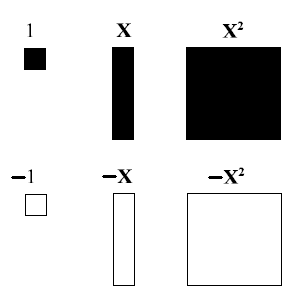# Using algebra tiles to factor polynomials - Introduction to Polynomials

### Using algebra tiles to factor polynomials

Algebra tiles can be used to represent polynomials. We can also use those tiles to solve polynomials. In this lesson, we will try to solve some polynomials using algebra tiles.

#### Lessons

##### Representing polynomials using algebra tiles: